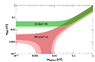# Majorana mass plot

• I
Malamala
Hello! In the attached plot, we have the Majorana mass as a function of the lightest neutrino for the normal (m1<m2<m3) and inverted (m3<m1<m2) hierarchy. Plugging in the values from the PMNS matrix in the formula for Majorana mass, I get something of the form: ##m_{\beta\beta} = 0.7m_1+0.25m_2+0.05m_3##. I am not sure I understand why do we have that downward branching for the normal hierarchy. Can someone explain it to me? Thank you!

#### Attachments

•majorana.png
10 KB · Views: 127

Mentor
What did you assume for the phase and did you try varying that a bit?

Malamala
What did you assume for the phase and did you try varying that a bit?
Thank you for your reply. For the angles I used the Wikipedia values. By phase I assume you mean the ##\delta_{CP}## parameter, right? If I understand it correctly, the formula for ##m_{\beta\beta}## contains only the first line of the PMNS matrix i.e. ##U_{e1}##, ##U_{e2}## and ##U_{e3}##. The phase appears only in ##U_{e3}##, but upon squaring that would cancel, wouldn't it? Is there another place where the phase appears?

Mentor
Squaring a complex number doesn't make the phase disappear, it just doubles the phase.
##m_{\beta\beta}## is the magnitude of the total complex sum, here is the formula taking this into account. An angle between 225 and 315 degrees (and between 45 and 135 degrees) leads to a square that has a negative real component.

Malamala
Squaring a complex number doesn't make the phase disappear, it just doubles the phase.
##m_{\beta\beta}## is the magnitude of the total complex sum, here is the formula taking this into account. An angle between 225 and 315 degrees (and between 45 and 135 degrees) leads to a square that has a negative real component.
Oh, I assumed that by squaring they meant ##aa^*##, now it makes more sense. So that phase leads to some sort of cancellation? It is still not clear to me why it is just in that mass range, and why it is not there at all in the inverted hierarchy case.

Mentor
You can plug in numbers and calculate.

In the normal order a small negative contribution from the heavy neutrino can cancel the rest. In the inverted order case you have two heavy masses and don't get that cancellation.